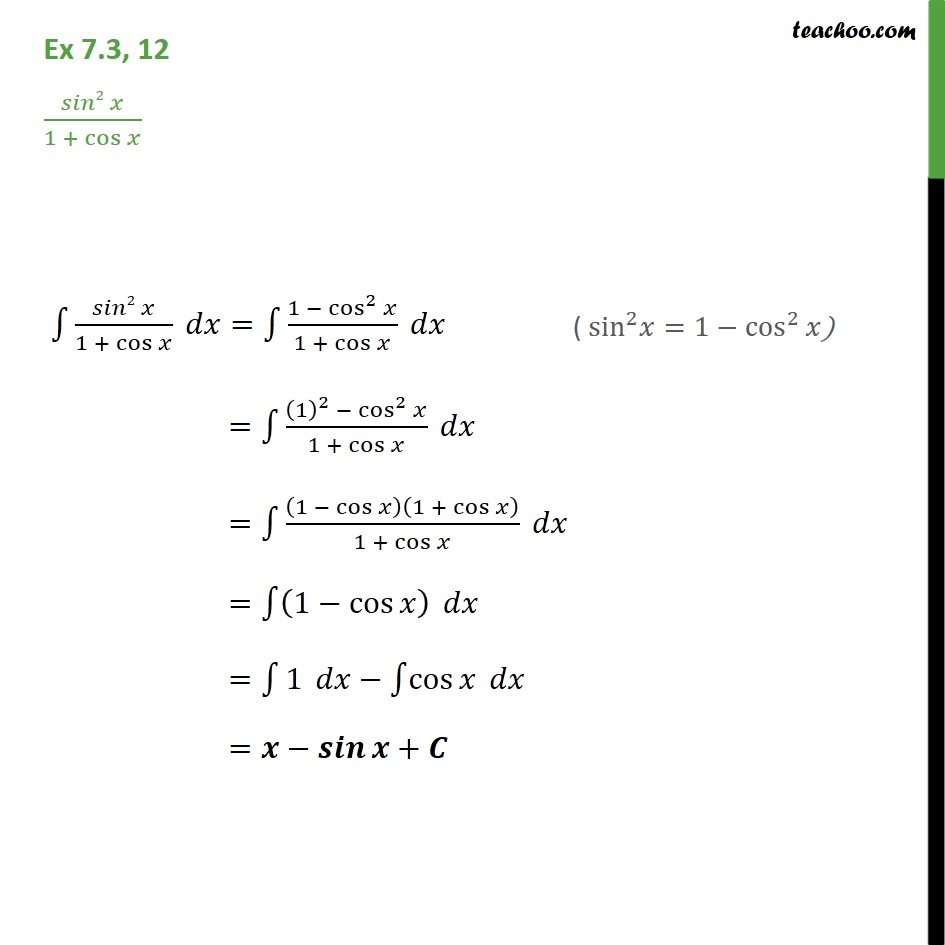Integration using trigo identities - sin^2,cos^2 etc formulae

Chapter 7 Class 12 Integrals
Concept wiseLearn in your speed, with individual attention - Teachoo Maths 1-on-1 Class

### Transcript

Ex 7.3, 12 ( 2 )/(1 + cos ) 1 ( 2 )/(1 + cos ) = 1 (1 cos^2 )/(1 + cos ) = 1 ((1)^2 cos^2 )/(1 + cos ) = 1 (1 cos )(1 + cos )/(1 + cos ) = 1 (1 cos ) = 1 1 1 cos = +